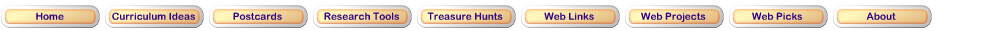Geometry Standard for Grades 3–5

Angles and Symmetry

Apply transformations and use symmetry to analyze mathematical situations
• predict and describe the results of sliding, flipping, and turning two-dimensional shapes;
• describe a motion or a series of motions that will show that two shapes are congruent;
• identify and describe line and rotational symmetry in two- and three-dimensional shapes and designs.Step 1View the Symmetry animation. It is a great visual introduction.

Step 2Visit these Websites and complete the activities.E-Lab Drag the line to the shapes to show the lines of symmetry.Sheppard Software Symmetry Game How many lines of symmetry does each shape have?Math Interactives Compose equivalent transformations in two different ways.AAA Math Polygons Identify the names of polygons by size and angle.Types of Triangles on a Geoboard Observe and label the angle as being acute, obtuse or right angle.Updated March 14, 2017
© 1996 - 2015 Linda C. Joseph